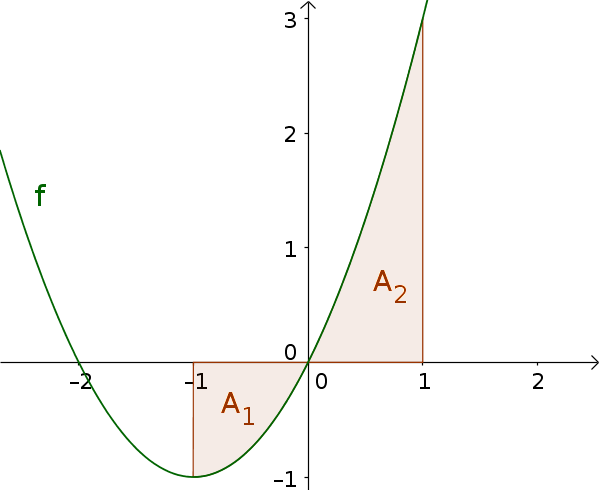Math Calculating areas with integrals Calculating areas by partitioning the interval

# Calculating areas by partitioning the interval

An exception to calculating areas are functions with sign changes in the interval $[a; b]$. This means that the area is partly above and partly below the x-axis.

!

### Remember

If the area you are looking for is both above and below the x-axis, you must calculate the areas separately.
This is the case when the function has a zero in the interval.
i

### Method

1. Determine zeros and find intervals
2. Calculate definite integrals for each interval
3. Determine area

### Example

Calculate the area between the graph of the function $f(x)=x^2+2x$ and the x-axis over the interval $[-1; 1]$1. #### Find intervals

First, calculate the zero(s) of the function.
$x^2+2x=0$
(Solve quadratic equation, e.g. remove brackets)
$x(x+2)=0$
$x_{N_1}=0$ and $x_{N_2}=-2$

$x_{N_1}=0$ is in the interval $[-1; 1]$. Therefore, the area must be divided into two parts:
$A_1$ over $[-1;0]$
$A_2$ over $[0;1]$
2. #### Find and calculate definite integrals

For both intervals, an integral must now be calculated.
$\int_a^b f(x) \, \mathrm{d}x$ $= [F(x) + C]_a^b$ $= F(b) - F(a)$
$F(x)=\frac13x^3+x^2$

$A_1$ over $[-1;0]$:
$\int_{-1}^0 (x^2+2x) \, \mathrm{d}x$ $= [\frac13x^3+x^2]_{-1}^0$ $= \frac13\cdot0^3+0^2 -$ $\frac13\cdot(-1)^3+(-1)^2$
$=0-\frac23$ $=-\frac23$

$A_2$ over $[0;1]$:
$\int_0^1 (x^2+2x) \, \mathrm{d}x$ $= [\frac13x^3+x^2]_0^1$ $= \frac13\cdot1^3+1^2 -$ $\frac13\cdot0^3+0^2$
$=\frac43-0$ $=\frac43$
3. #### Determine area

Now the area of each partition has to be determined and then added.
$A_1=|\int_{-1}^0 f(x)\,\mathrm{d}x|$ $=|-\frac23|$ $=\frac23$
$A_2=\int_0^1 f(x)\,\mathrm{d}x$ $=\frac43$

$A=A_1+A_2$ $=\frac23+\frac43=2$